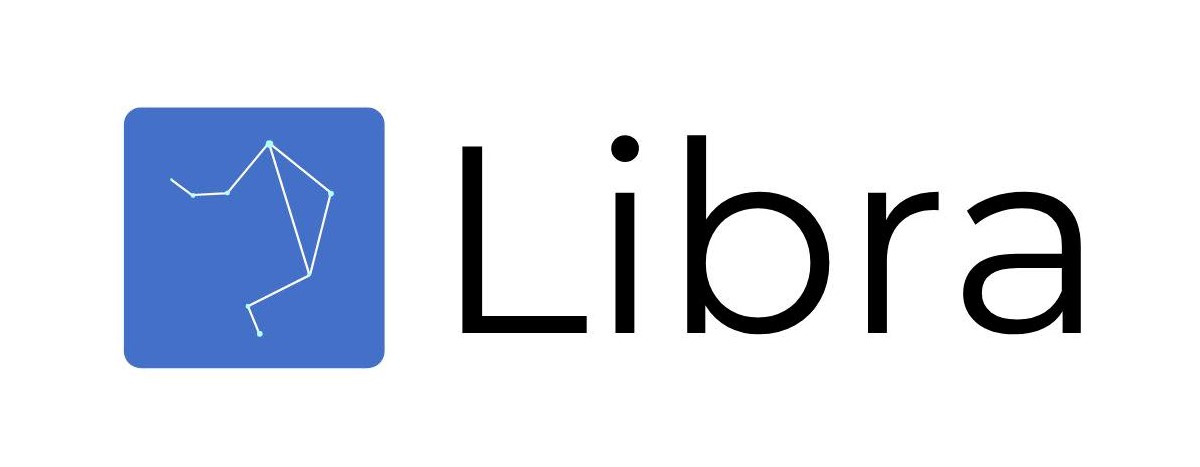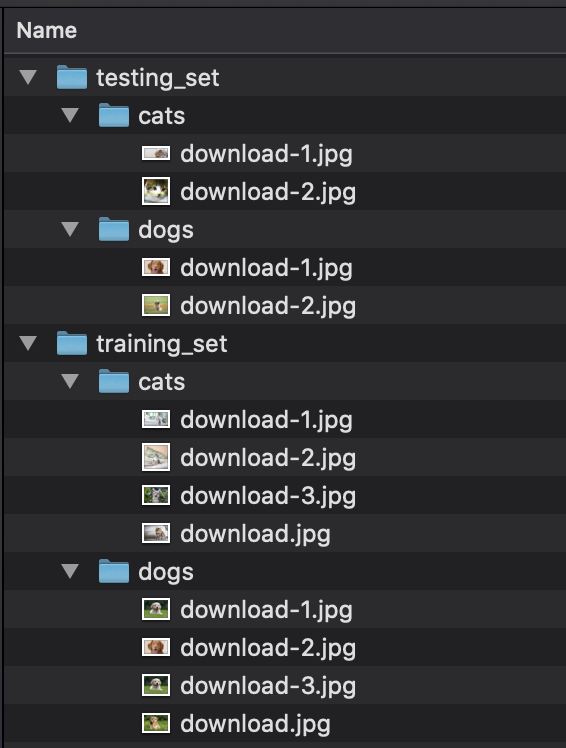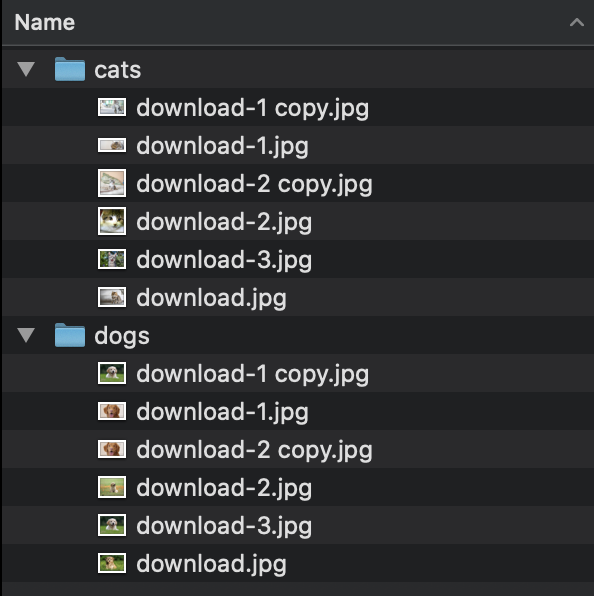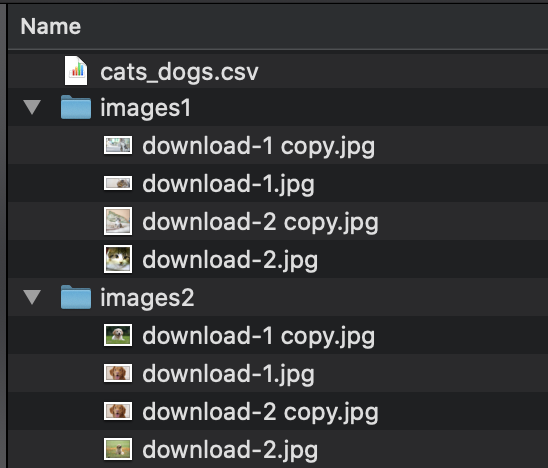## General Dataset Guidelines

These instructions apply to all of the modeling queries EXCEPT convolutional_query()

• ONLY the instruction parameter is required for the queries.
• When providing your instruction make sure that you're describing a column. For example: if you want the dataset to use a column named median_house_value as the target, make your instruction "please estimate the median house value". If you want your target to be number_of_households, make your instruction "In Baltimore, model the number of households".
• You can also just pass the column name in as the instruction
• ALL tabular datasets will work with this query. The more parameters you specify, the more optimized your model will be.

# neural_network_query()

Automatically fits a neural network to your dataset. Target detection, preprocessing, and scoring are done by default.

 Arguments instruction=None An English language statement that represents the task you would like to be completed. eg: 'predict the median house value' or 'please estimate the number of households'. Should correspond to a column in the dataset. ca_threshold=0.2 Changes the proportion at which correspondence analysis is applied. This deals with reducing the number of categorical features that are one-hot-encoded. drop=[] Columns to drop manually, drop columns with links, weirdly formatted numbers, and others. text=[] Represents any columns that contain long pieces of text to be embedded into vectors. preprocess=True Whether you want your dataset to be intelligently preprocessed test_size=0.2 The proportion of your entire dataset that is used for testing. random_state=49 The state ticker you want to be set at. epochs=50 Number of epochs. This is for every model that's created in the process. generate_plots=True Whether you want libra to create accuracy and loss plots for you. save_model=False Whether the model is saved in a .json and .h5 file. save_path='current_directory' Where do you want the save_model information to be stored. Default is current working directory.
```new_client = client('path_to_dataset')
new_client.neural_network_query('Please estimate the number of households.')
new_client.models['regression_ANN'].plots() #access plots```

# convolutional_query()

Automatically fits a Convolutional Neural Network to your dataset. Images are automatically interpolated to the median height and wiedth. Three types of dataset structures are supported. Read below for more information.

 Arguments instruction=None An English language statement that represents the task you would like to be completed. eg: 'predict the median house value' or 'please estimate the number of households'. Should correspond to a column in the dataset. Only neccesary if read_mode is 'csvwise'. image_column=None Only used when read_mode is ‘csvwise’. The column title containing the images. generate_plots=True Whether you want libra to create accuracy and loss plots for you. read_mode=distinguisher() Identifies the format in which data the present in. If none is specified then the query will automatically identify the format of your data. Note that only three types of formats are currently supported (listed in the table below). new_folders=True This query will automatically crop all images to an intelligent size and create new folders with these images. If you would like it to replace your original images then set this to false. image_column=None If you're using pathwise from a csv, you can by default use a column in the set as the path location. test_size=0.2 Represents the size of the testing set. preprocess=True Whether you want your dataset to be intelligently preprocessed. augmentation=True Magnifies dataset to add small variation to images before training in the model. epochs=10 Number of epochs for every model attempted. height=None Resizes all images to the given height. If left as None, will resize images’ to the median height. width=None Resizes all images to the given width. If left as None, will resize images’ to the median width. verbose=0 Whether inner computation is displayed. This will spam the console box. custom_arch=None Whether inner computation is displayed. This will spam the console box. save_as_tfjs=False Save trained model as a TensorFlow.js file. save_as_tflite=False Save trained model as a Tensorflow.lite file. Can be used for lighter deployments. pretrained=None Load a state-of-the-art model architecture through dictionary with keys 'arch' and 'weights'. Current values supported for 'arch' key are 'vggnet16', 'vggnet19', 'resnet50', 'resnet101', 'resnet152', 'mobilenetv2', 'densenet121', 'densenet169', and 'densenet201'. Possible values for 'weights' key include 'imagenet' and 'random'.
```newClient = client('path_to_directory_with_image_folders')
newClient.convolutional_query("Please classify my images", pretrained={'arch':'vggnet19', 'weights':'imagenet'})```

 Read Modes 'setwise' Directory consists of a ‘training_set’ and ‘testing_set’ folder both containing classification folders with images inside.'classwise' Directory consists of classification folders with images inside.'csvwise' Directory consists of image folders and a CSV file that has an image column.# svm_query()

Automatically fits a support vector machine to your dataset. Only classification is supported. SVRegressor will be coming soon. Target detection, preprocessing, and scoring are done by default.

 Arguments instruction An English language statement that represents the task you would like to be completed. eg: 'predict the median house value' or 'please estimate the number of households'. Should correspond to a column in the dataset. test_size=0.2 The proportion of your entire dataset that is used for testing. text=[] Represents any columns that contain long pieces of text to be embedded into vectors. random_state=49 The state ticker you want to be set at kernel='linear' The type of kernel trick you want to be used. Options include 'rbf' and 'poly'. preprocess=True Whether you want your dataset to be intelligently preprocessed drop=[] Columns to drop manually, drop columns with links, weirdly formatted numbers, and others. degree=3 Degree of the polynomial kernel function. Only used by 'poly' kernel. gamma='scale' Kernel coefficient for 'rbf', 'poly' and 'sigmoid'. coef0=0.0 Independent term in kernel function. It is only significant in ‘poly’ and ‘sigmoid’. max_iter=1 Hard limit on iterations within solver, or -1 for no limit.
```newClient = client('path_to_file')
newClient.svm_query('Model the type of credit card')```

# nearest_neighbor_query()

Automatically fits a KNN to your dataset. Target detection, preprocessing, and scoring are done by default.

 Arguments instruction An English language statement that represents the task you would like to be completed. eg: 'predict the median house value' or 'please estimate the number of households'. Should correspond to a column in the dataset. test_size=0.2 The proportion of your entire dataset that is used for testing. text=[] Represents any columns that contain long pieces of text to be embedded into vectors. random_state=49 The state ticker you want to be set at preprocess=True Whether you want your dataset to be intelligently preprocessed. drop=[] Columns to drop manually, drop columns with links, weirdly formatted numbers, and others. min_neighbors=3 The minimum number of nearest neighbors to calculate the loss with. max_neighbors=3 The maximum number of nearest neighbors to calculate the loss with. leaf_size=30 Leaf size passed to BallTree or KDTree. This can affect the speed of the construction and query, as well as the memory required to store the tree. p=2 Parameter for the Minkowski metric from sklearn.metrics.pairwise.pairwise_distances. When p = 1, this is equivalent to using manhattan_distance (l1), and euclidean_distance (l2) for p = 2. algorithm='auto' Algorithm used to compute the nearest neighbors: ‘ball_tree’ will use BallTree, ‘kd_tree’ will use KDTree, ‘brute’ will use a brute-force search, ‘auto’ will attempt to decide the most appropriate algorithm based on the values passed to fit method.
```newClient = client('path_to_file')
newClient.nearest_neighbors_query('Model the type of credit card')```

# decision_tree_query()

Automatically fits a decision tree algorithm to your dataset. Target detection, preprocessing, and scoring are done by default.

 Arguments instruction An English language statement that represents the task you would like to be completed. eg: 'predict the median house value' or 'please estimate the number of households'. Should correspond to a column in the dataset. preprocess=True Whether you want your dataset to be intelligently preprocessed. test_size=0.2 The proportion of your entire dataset that is used for testing. text=[] Represents any columns that contain long pieces of text to be embedded into vectors. drop=[] Columns to drop manually, drop columns with links, weirdly formatted numbers, and others. criterion='gini' The function to measure the quality of a split. Supported criteria are “gini” for the Gini impurity and “entropy” for the information gain. splitter='best' The strategy used to choose the split at each node. Supported strategies are “best” to choose the best split and “random” to choose the best random split. max_depth=None The maximum depth of the tree. If None, then nodes are expanded until all leaves are pure or until all leaves contain less than min_samples_split samples. min_samples_split=2 The minimum number of samples required to split an internal node. min_weight_fraction_leaf=0.0 The minimum weighted fraction of the sum total of weights (of all the input samples) required to be at a leaf node. max_leaf_nodes=None Grow a tree with max_leaf_nodes in best-first fashion. Best nodes are defined as relative reduction in impurity. min_impurity_decrease=0.0 A node will be split if this split induces a decrease of the impurity greater than or equal to this value. ccp_alpha=0.0 Complexity parameter used for Minimal Cost-Complexity Pruning. The subtree with the largest cost complexity that is smaller than ccp_alpha will be chosen.
```newClient = client('path_to_file')
```newClient = client('path_to_file')